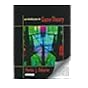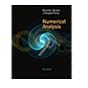Normal view MARC view ISBD view

# Numerical analysis / Richard L. Burden, J. Douglas Faires.

Material type:BookPublisher: Boston, MA : Brooks/Cole, Cengage Learning, c2011Edition: 9th ed.Description: xiv, 872 p. : col. ill. 27 cm.ISBN: 9780538733519; 0538733519; 9788131516546.Subject(s): Numerical analysisDDC classification: 519.4
Contents:
Mathematical preliminaries and error analysis -- Solutions of equations in one variable -- Interpolation and polynomial approximation -- Numerical differentiation and integration -- Initial-value problems for ordinary differential equations -- Direct methods for solving linear systems -- Iterative techniques in matrix algebra -- Approximation theory -- Approximating eigenvalues -- Numerical solutions of nonlinear systems of equations -- Boundary-value problems for ordinary differential equations -- Numerical solutions to partial differential equations.
List(s) this item appears in: Weekly Addition
Tags from this library: No tags from this library for this title.
Item type Current location Collection Call number Status Date due BarcodeGeneral Books

This is a searchable open catalogue of all library of the Central University of Tamil Nadu.

Sciences
Non-fiction 519.4 BUR (Browse shelf) Available 37690
##### Browsing CUTN Central Library Shelves , Shelving location: Sciences , Collection code: Non-fiction Close shelf browser519.3 OSB On Introduction to Game Theory 519.3 OSB On Introduction to Game Theory 519.3 OSB On Introduction to Game Theory 519.4 BUR Numerical analysis / 519.5 Life Insurance Mathematics 519.5 ACZ Complete business statistics 519.5 ACZ Complete business statistics

Mathematical preliminaries and error analysis -- Solutions of equations in one variable -- Interpolation and polynomial approximation -- Numerical differentiation and integration -- Initial-value problems for ordinary differential equations -- Direct methods for solving linear systems -- Iterative techniques in matrix algebra -- Approximation theory -- Approximating eigenvalues -- Numerical solutions of nonlinear systems of equations -- Boundary-value problems for ordinary differential equations -- Numerical solutions to partial differential equations.

Includes bibliographical references (p. 763-772) and index.

There are no comments for this item.